2022-01-30 00:16:38

Hello! , I'm Manon Feige , Thank you for reading this article , Welcome to three links with one button . Further introduction Pillow Library usage , Learn more about Dry cargo is full. , Recommended collection , I need to use it. I need to look at it from time to time . If you have any questions and need , Welcome to leave a message ~ ~ ~.

## Preface

This article is a continuation of the previous one ️【Python From entry to mastery 】（ hexacosa- ） use Python Of PIL library （Pillow） Processing images is really handy ️ Further introduction Pillow Library usage , This article will focus on some advanced features ： For example, how to use Pillow Draw a picture （ circular , Square ）, Introduced by Pillow Add a watermark to the picture ; At the same time, the common knowledge points not introduced in the previous article are supplemented . I hope it can help readers .

## Image modular

The last article introduced Image modular , But the introduction is not comprehensive enough , For example, how to read pictures from web pages is not introduced to , How to cut the picture is not introduced .

### Read the pictures in the web page

The basic implementation of reading pictures in web pages is ： The first use of requests The library reads the contents of the current picture link , Then convert the content into binary data , Through open Method to convert the binary data , Finally through save Method to save .

``````from PIL import Image
from io import BytesIO
import requests
res = requests.get(
# Convert the picture content to binary
im2 = Image.open(BytesIO(res))
b = BytesIO()
im2.save(b, format='PNG')
im2.show()
Copy code ``````

The reading result is ：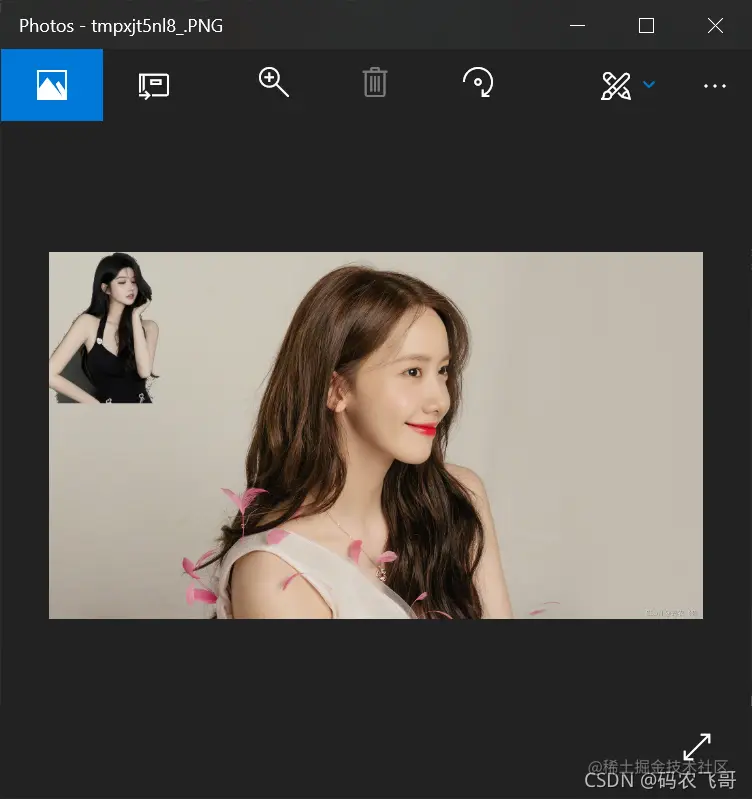### Image clipping

adopt crop Method to crop an area of a specified size from the picture . The cut area is `(left, upper, right, lower)` For example, from a certain width and height 400 The width and height of one cut in the picture are 100 The square area of , You only need to specify that the coordinates of the clipping region are : `(0, 0, 100, 100)`

``````im2 = Image.new('RGBA', (400, 400), 'blue')
box = (0, 0, 100, 100)
region = im2.crop(box)  #  Set the region to crop
region.show()
Copy code ``````

There is also a way to clip, which is to resize the picture resize, For example, put the front 400400 Pictures of the Modified into 300200, Just call resize Method

``````img4 = im2.resize((300, 200))
img4.show()
Copy code ``````

### Description of picture mode ：

Pattern describe
1 1 Bitpixel , Black and white image , Deposit 8 Bitpixel
L 8 Bitpixel , Black and white
P 8 Bitpixel , Use the palette to map to any other mode
RGB 3*8 Bitpixel , True color
RGBA 4*8 Bitpixel , True color + Transparent channel
CMYK 4*8 Bitpixel , Four color printing mode or color printing mode
YCbCr 3*8 Bitpixel , Color video format
I 32 Bit integer pixels
F 33 Bit floating point pixels

adopt convert Method to convert the picture mode

## ImageDraw modular

Previously introduced ImageDraw library , It just introduces how to use it to write text to pictures , Actually ImageDraw There is a more useful way to use modules , It can be used to draw all kinds of graphics .

### The method of drawing

Method demonstration effect
ImageDraw.line(xy, fill=None, width=0, joint=None) draw.line([100, 100, 100, 500], fill='blue', width=2) Draw a square
ImageDraw.arc(xy, start, end, fill=None, width=1) draw.arc([100, 100, 600, 600], 0, 360, fill='black') Draw an arc
ImageDraw.ellipse(xy, fill=None, outline=None, width=1) draw.ellipse([100, 100, 600, 600], outline='black', fill='white') A circle
ImageDraw.chord(xy, start, end, fill=None, outline=None, width=1) draw.chord([100, 100, 600, 600], 0, 360, outline=125) Draw a semicircle , Be similar to arc()
ImageDraw.pieslice(xy, start, end, fill=None, outline=None, width=1) draw.pieslice([100, 100, 600, 600], 180, 210, outline=255) Draw fan
ImageDraw.rectangle(xy, fill=None, outline=None, width=1) draw.rectangle((200, 200, 500, 500), outline='yellow') Draw a rectangular
ImageDraw.rounded_rectangle(xy, radius=0, fill=None, outline=None, width=1) draw.rounded_rectangle((200, 200, 600, 600), radius=1, outline='green') Draw a rounded rectangle （ Or square ）

### Draw a square

First create a 600*600 Canvas of . Then draw a square on the canvas , The way to draw a straight line is line Method . ImageDraw.line(xy, fill=None, width=0, joint=None)

stay xy Draw a straight line between the coordinates of xy--> Draw a straight line between two coordinate points , The input mode of coordinate points is [(x, y), (x, y), ...] perhaps [x, y, x, y, ...] fill--> Color of line width--> The width of the line

``````#  Draw a dot
im = Image.new('RGB', (600, 600), 'white')
draw = ImageDraw.Draw(im)

# #  Create a square ,fill  It's color
draw.line([100, 100, 100, 500], fill='blue', width=2)  #  Left vertical line
draw.line([100, 100, 500, 100], fill='blue', width=2)  #  Upper horizontal line
draw.line([500, 100, 500, 500], 'blue', width=2) # Right vertical line
draw.line([100, 500, 500, 500], 'blue', width=2) # Bottom horizontal line
im.save('picture/sequare.png', 'png')
im.show()
Copy code ``````

Draw a border with a width of 2px, The color is blue , Area is 400*400 The square of .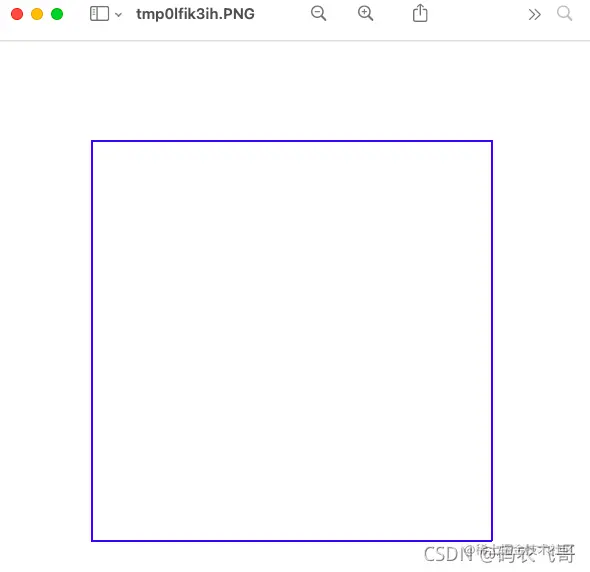### Draw an arc

ImageDraw.arc(xy, start, end, fill=None, width=0)

Within a given area , Draw an arc from the start angle to the end angle xy--> Define the two points of the bounding box , The incoming format is [`(x0, y0), (x1, y1)]` perhaps `[x0, y0, x1, y1]` , among x1>=x0,y1>=y0 start --> Starting angle , In degrees , from 3 It starts at o'clock and increases clockwise end--> End angle , In degrees fill--> The color of the arc width--> The width of the arc

``````draw.arc([100, 100, 600, 600], 0, 180, fill='black')
im.show()
Copy code ``````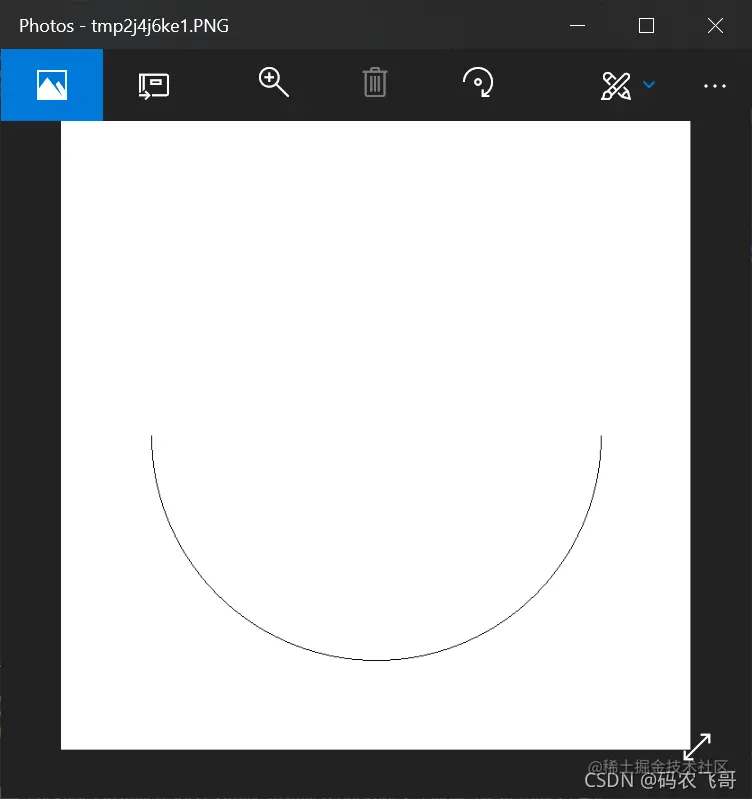Here is a semicircle , If the end angle is 360 Degrees will draw a complete circle .

### A circle

Draw a circle through ImageDraw.ellipse(xy, fill=None, outline=None, width=1) Method , This method can draw a circle in a given range

xy--> Define the two points of the bounding box , The incoming format is [`(x0, y0), (x1, y1)]` perhaps `[x0, y0, x1, y1]` , among x1>=x0,y1>=y0 outline--> Color of outline fill ---> Fill color width--> The width of the outline

``````draw.ellipse([100, 100, 600, 600], outline='black', fill='blue')
im.show()
Copy code ``````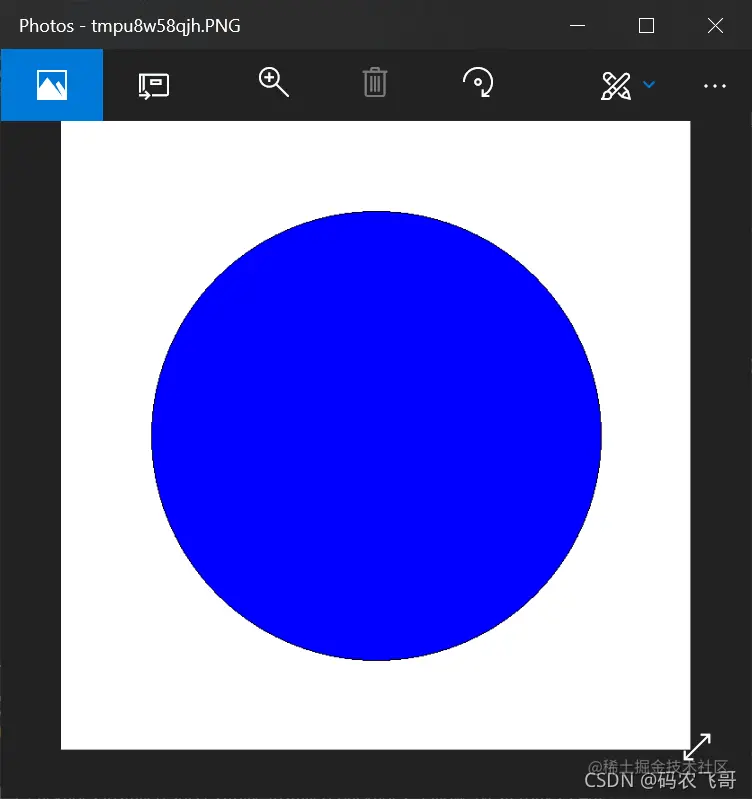### Draw a semicircle

ImageDraw.chord(xy, start, end, fill=None, outline=None, width=1) Method is used to draw a semicircle , Follow arc() The difference is that it connects the start point and the end point with a straight line

xy--> Define the two points of the bounding box , The incoming format is [`(x0, y0), (x1, y1)]` perhaps `[x0, y0, x1, y1]` , among x1>=x0,y1>=y0 outline--> Color of outline fill ---> Fill color width--> The width of the outline

``````draw.chord([100, 100, 600, 600], 0, 180,  outline='black', fill='red')
im.show()
Copy code ``````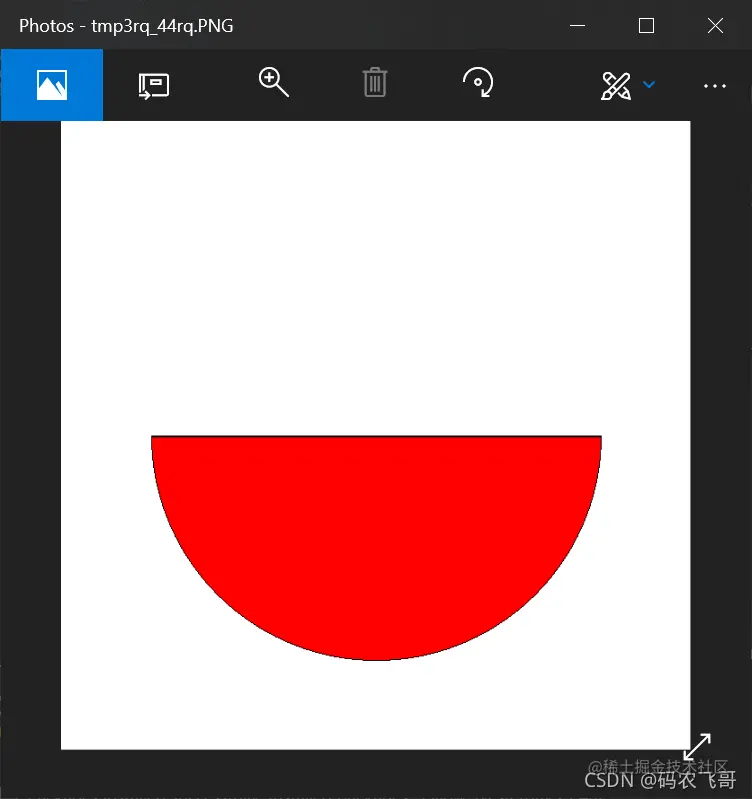### Draw fan

ImageDraw.pieslice(xy, start, end, fill=None, outline=None, width=1) Be similar to arc() Method , But he will draw a straight line between the endpoint and the dot xy--> Define the two points of the bounding box , The incoming format is [`(x0, y0), (x1, y1)]` perhaps `[x0, y0, x1, y1]` , among x1>=x0,y1>=y0 start --> Starting angle , In degrees , from 3 It starts at o'clock and increases clockwise end--> End angle , In degrees fill--> The color of the arc width--> The width of the arc

``````draw.pieslice([100, 100, 600, 600], 180, 300, outline='red', fill='blue')
im.show()
Copy code ``````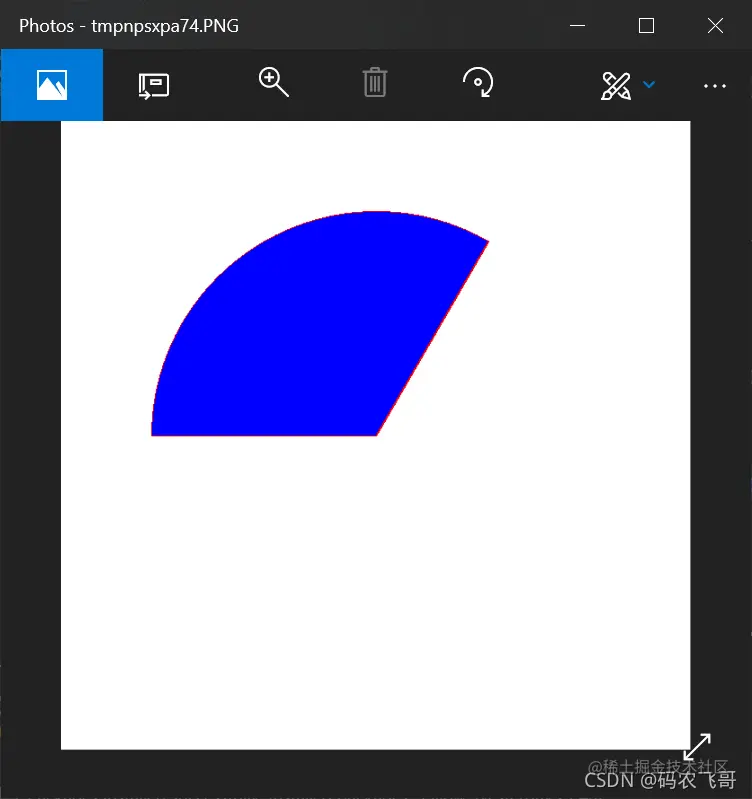## Draw a rectangular

ImageDraw.rectangle(xy, fill=None, outline=None, width=1) xy--> Draw a straight line between two coordinate points , The input mode of coordinate points is [(x, y), (x, y), ...] perhaps [x, y, x, y, ...] outline--> Color of outline fill--> Fill color width--> The width of the outline

``````#  rectangular
draw.rectangle((100, 200, 300, 500), outline='red', fill='blue')
im.show()
Copy code ``````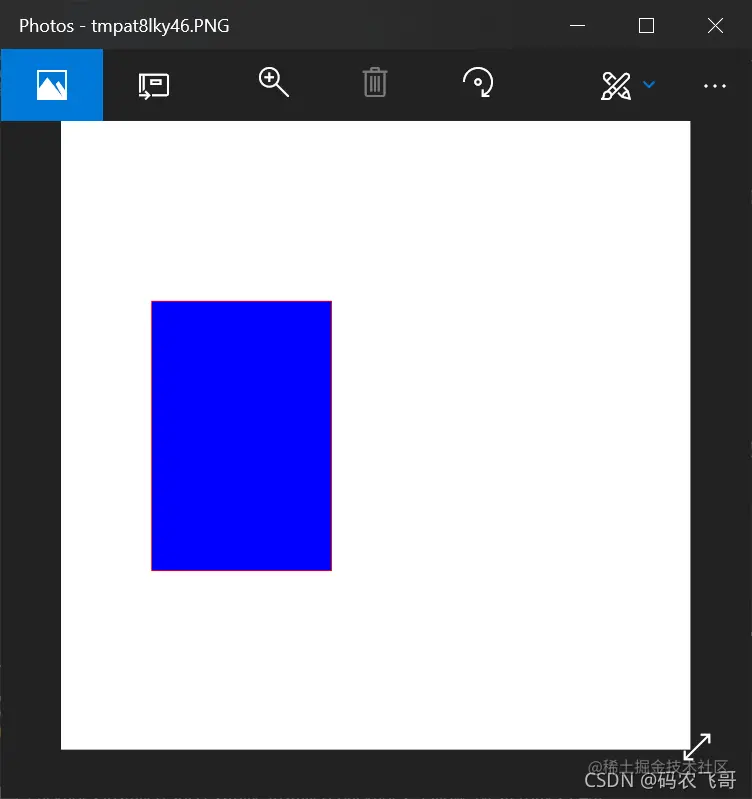### Draw a rounded rectangle

ImageDraw.rounded_rectangle(xy, radius=0, fill=None, outline=None, width=1) This method can draw a rounded rectangle xy--> Draw a straight line between two coordinate points , The input mode of coordinate points is [(x, y), (x, y), ...] perhaps [x, y, x, y, ...] radius--> The radius of the corner outline--> Color of outline fill--> Fill color width--> The width of the outline

``````draw.rounded_rectangle((200, 200, 600, 600), radius=2, outline='green')
im.show()
Copy code ``````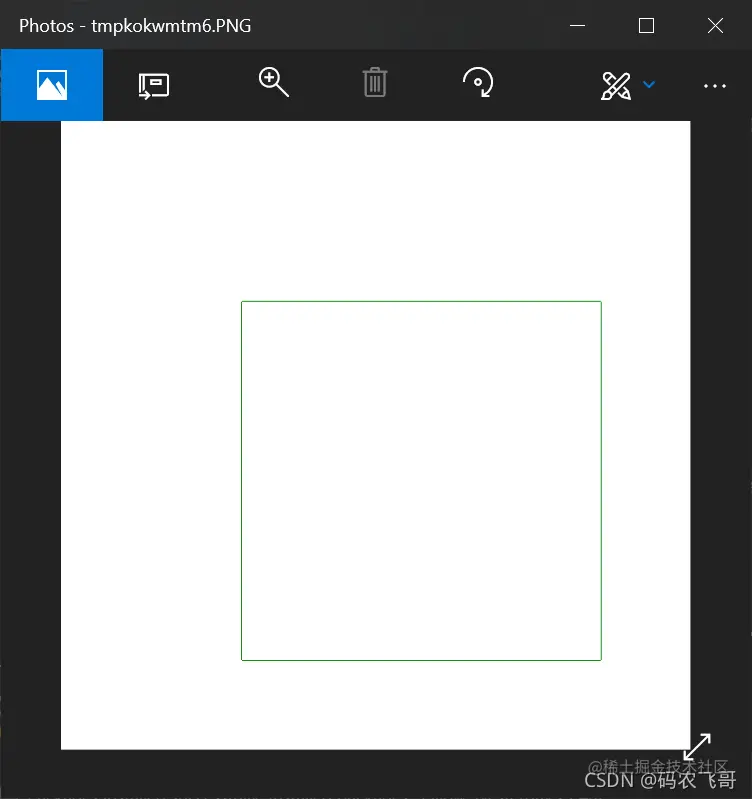## Solve a problem

Here's the problem , Is how to draw a good figure from Image Buckle it out ？

## ImageEnhance modular

ImageEnhance The module is mainly used to set the color, contrast, brightness and sharpness of the picture , Enhance the image .

1. Original picture
``````from PIL import ImageEnhance, Image

org_img = Image.open('picture/img10.png')
org_img.show(title=' Original picture ')
Copy code ``````

original image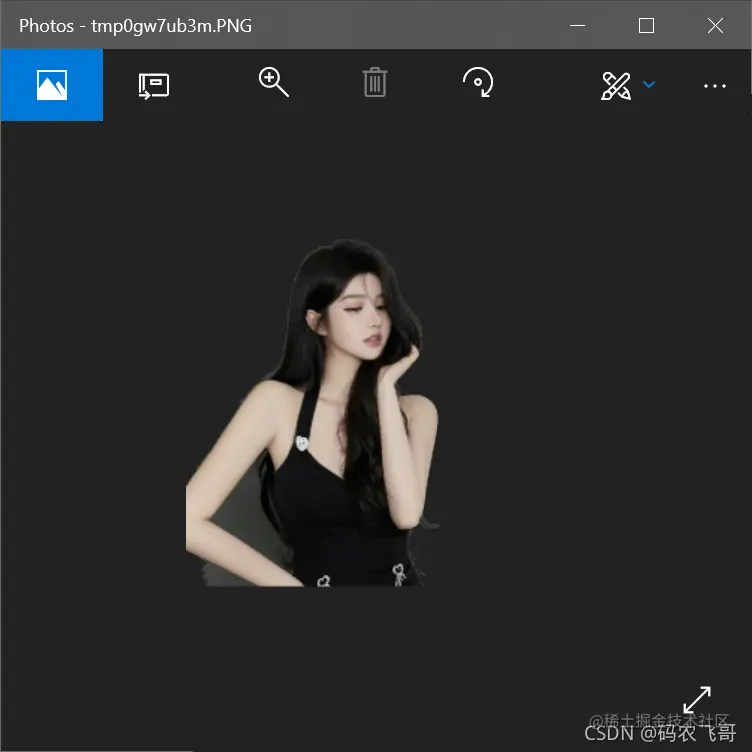1. Color balance of the image , Color enhancement 1.2 times

PIL.ImageEnhance.Color(image) Method , This method is mainly used to adjust the color balance of the image , The coefficient of the original image is 1.0,0.0 The enhancement coefficient is a black-and-white image

``````cl = ImageEnhance.Color(org_img)
# Black and white image
ce = cl.enhance(0)
ce.show()
# enhance 1.2 times
ce1 = cl.enhance(1.2)
ce1.show()
Copy code ``````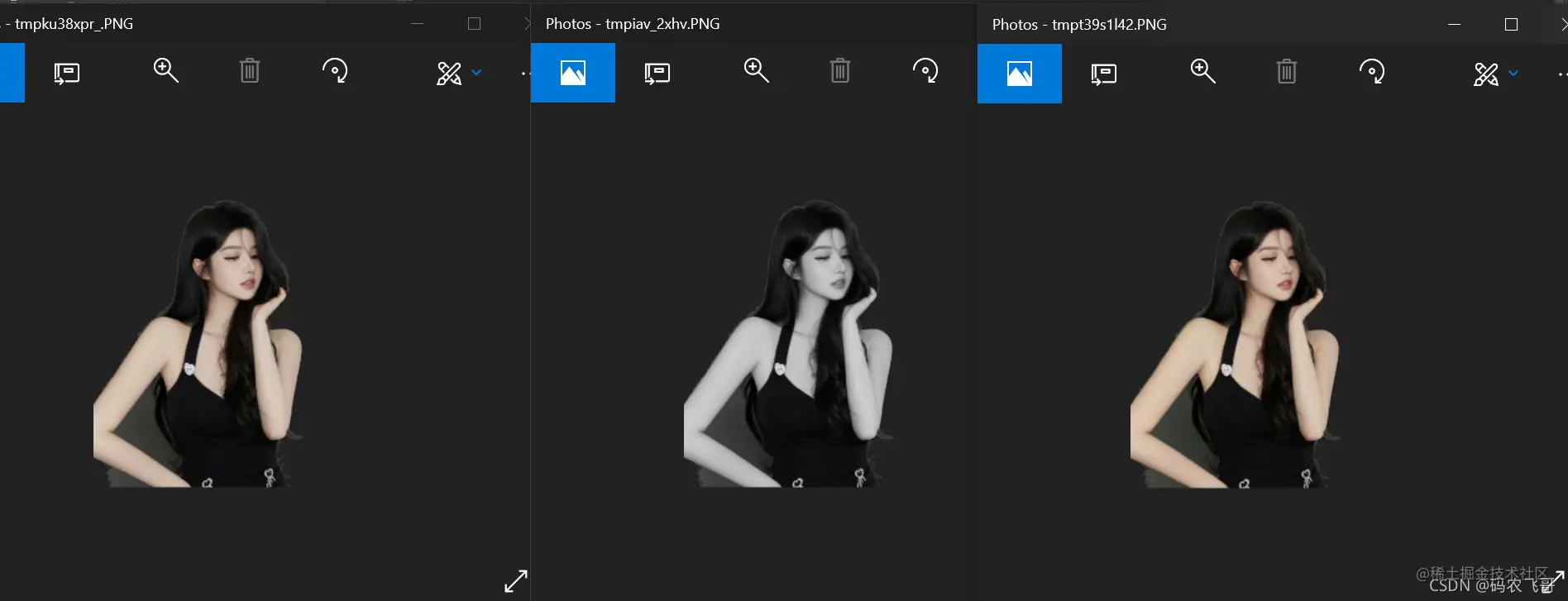1. Adjust the contrast of the image 3:4

PIL.ImageEnhance.Contrast(image) This method is mainly used to adjust the contrast of the image , Similar to contrast control on TV ,0.0 The enhancement coefficient gives a pure gray image , coefficient 1.0 Then the original image

``````ct = ImageEnhance.Contrast(org_img)
ch = ct.enhance(0)
ch.show(title=' Contrast 0 Pure grey ')
ch1 = ct.enhance(3.4)
ch1.show(title=' Contrast 3.4')
Copy code ``````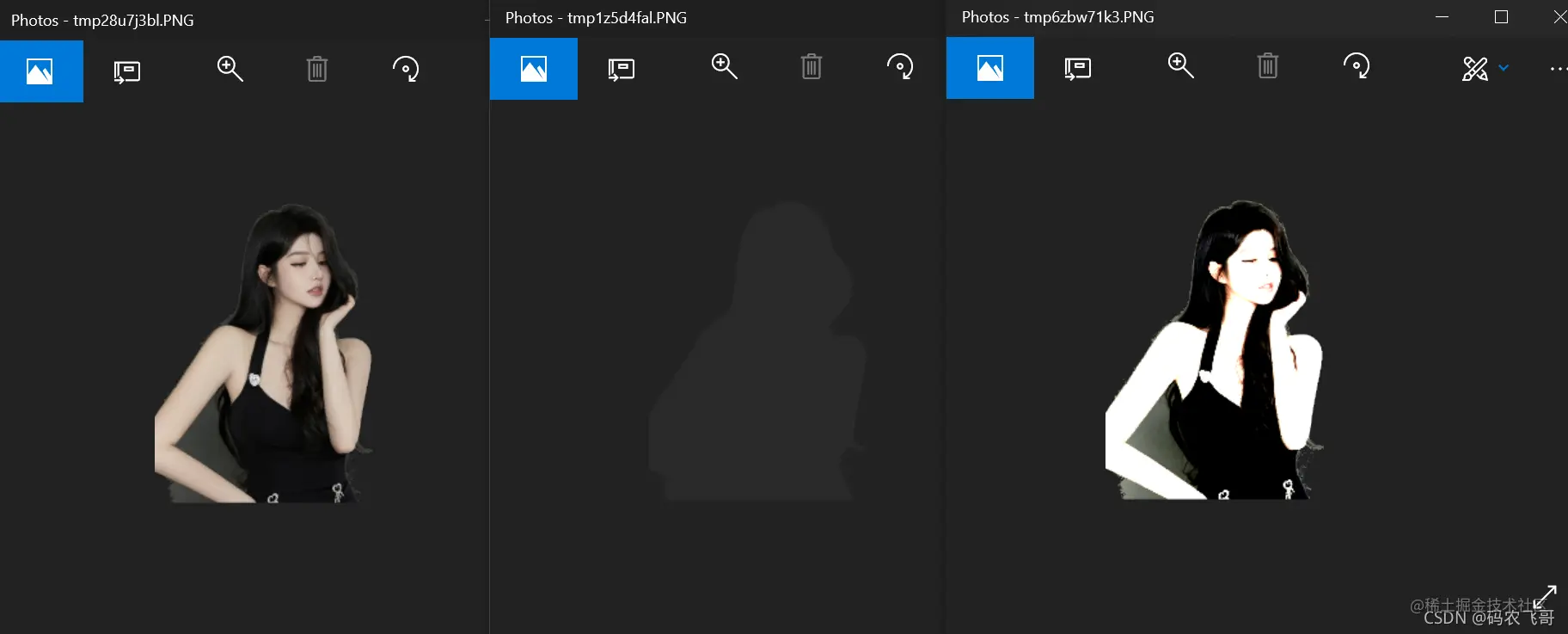7. Adjust the brightness of the image PIL.ImageEnhance.Brightness(image) , This method is mainly used to adjust the brightness of the image ,0.0 The enhancement coefficient of represents the black image . The coefficient is 1.0 Then the original image .

``````br = ImageEnhance.Brightness(org_img)
be = br.enhance(0)
be.show(title=' brightness 0')
be = br.enhance(3)
be.show(title=' brightness 3')
Copy code ``````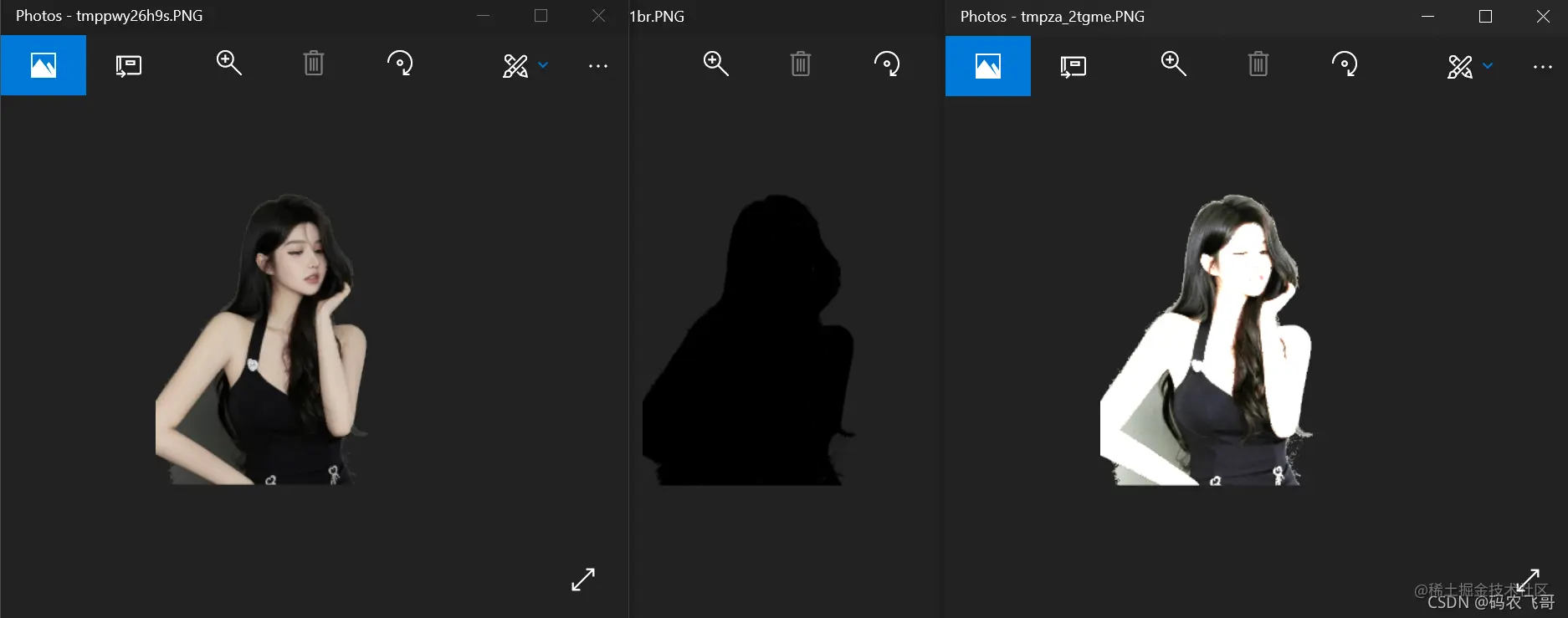1. Adjust the sharpness of the image PIL.ImageEnhance.Sharpness(image) , This method is mainly used to adjust the sharpness of the image ,0.0 The enhancement factor is blurred image ,1.0 The enhancement factor is the original image ,2.0 The enhancement factor is sharpened image .
``````sp = ImageEnhance.Sharpness(org_img)
se = sp.enhance(0)
se.show(title=' Sharpness 0')
se1 = sp.enhance(20)
se1.show(title=' Sharpness 20')
Copy code ``````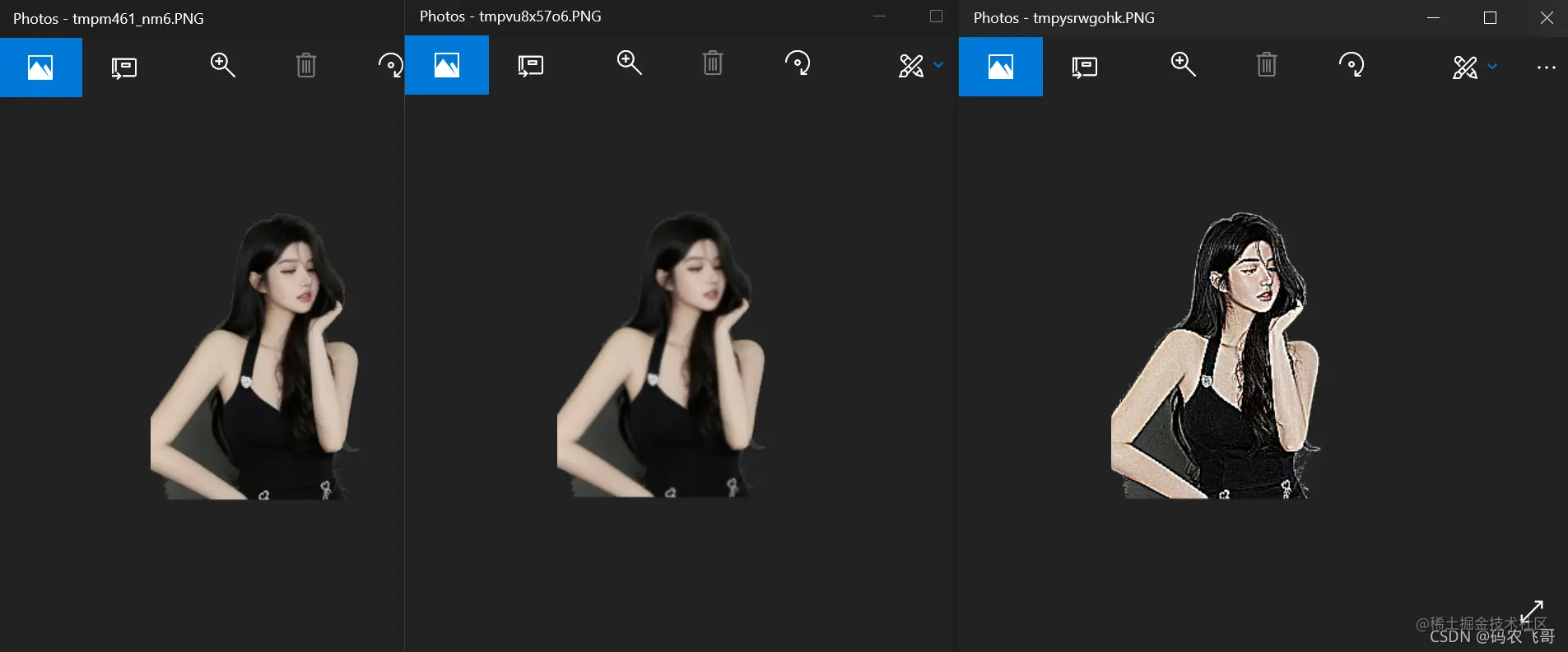## ImageFilter modular

ImageFilter The module is mainly used to filter the image , Enhance edges , blurred , The use of this module is `im.filter(ImageFilter)` . among ImageFilter Pass in the specified filter value as required .

Filter value effect
ImageFilter.GaussianBlur Gaussian blur
ImageFilter.BLUR Ordinary blur
ImageFilter.EDGE_ENHANCE Edge enhancement
ImageFilter.FIND_EDGES Find the edge
ImageFilter.EMBOSS Relief
ImageFilter.CONTOUR outline
ImageFilter.SHARPEN sharpening

Let's try the effect one by one

1. Original picture
``````from PIL import Image, ImageFilter
im = Image.open('picture/img10.png')
im.show()
Copy code ``````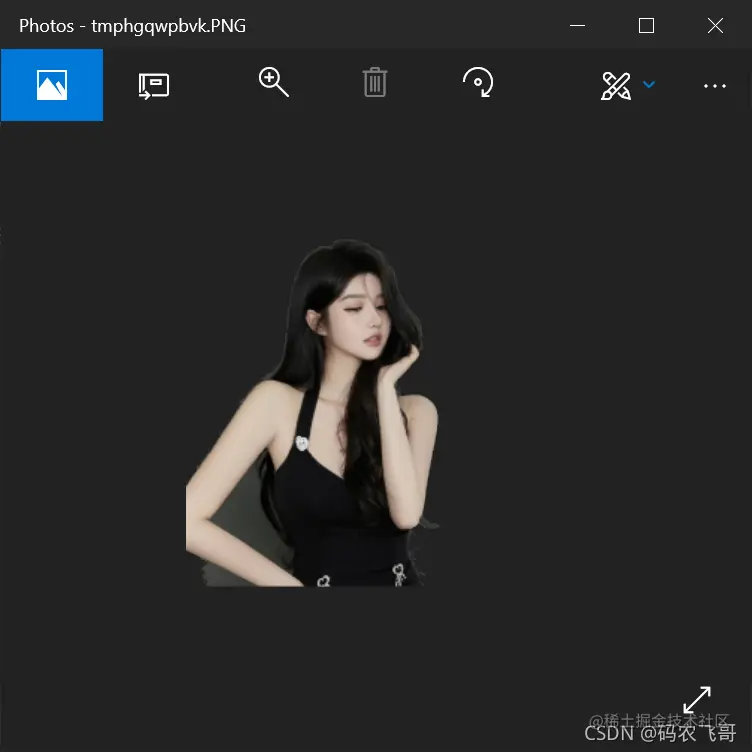2. Gaussian blur

``````im1 = im.filter(ImageFilter.GaussianBlur)
im1.show()
Copy code ``````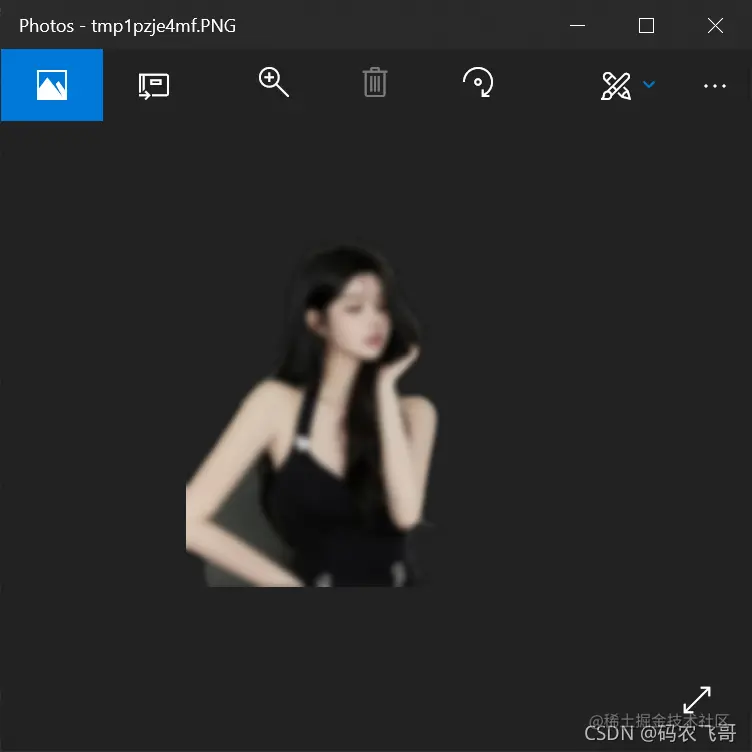3. Ordinary blur

``````im2 = im.filter(ImageFilter.BLUR)
im2.show()
Copy code ``````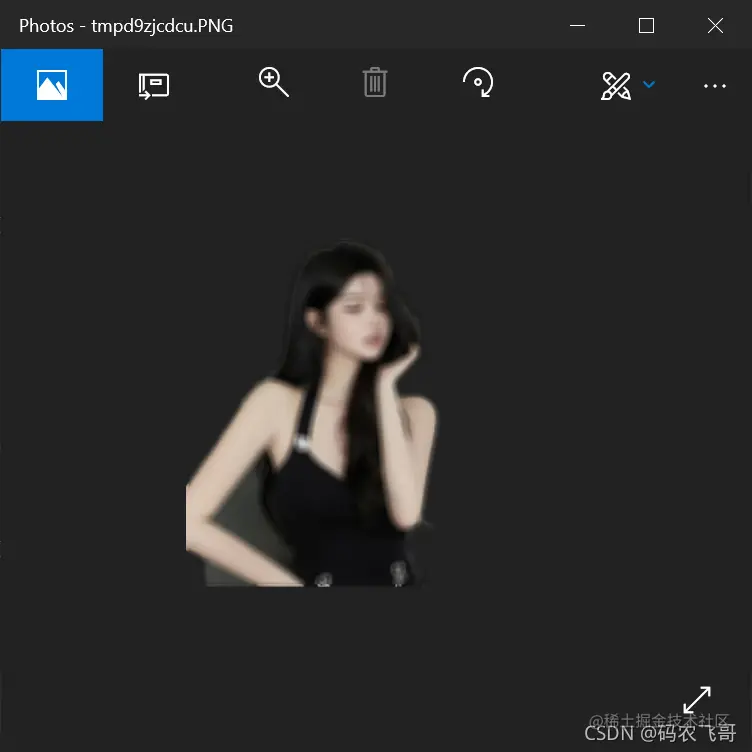4. Edge enhancement

``````im3 = im.filter(ImageFilter.EDGE_ENHANCE)
im3.show()
Copy code ``````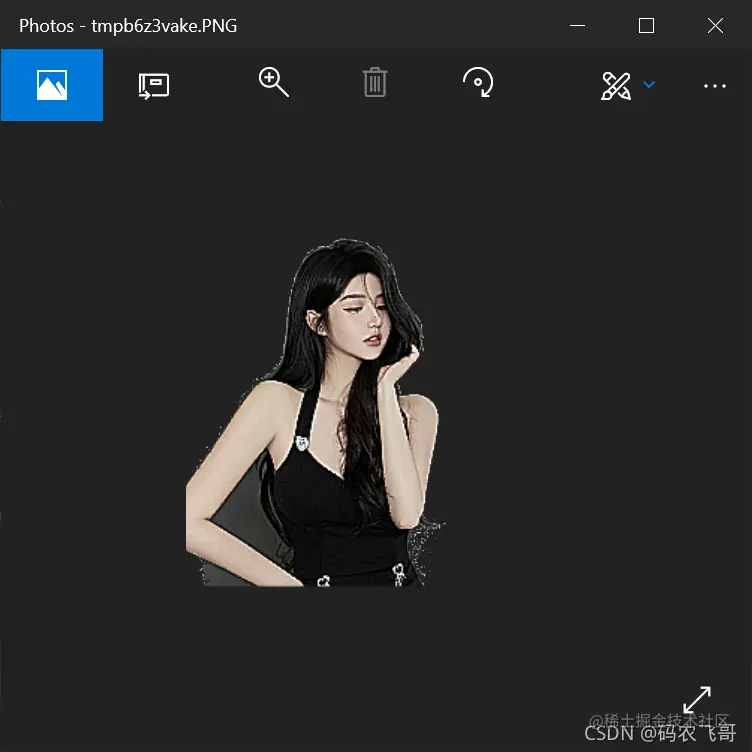5. Find the edge

``````im4 = im.filter(ImageFilter.FIND_EDGES)
im4.show()
Copy code ``````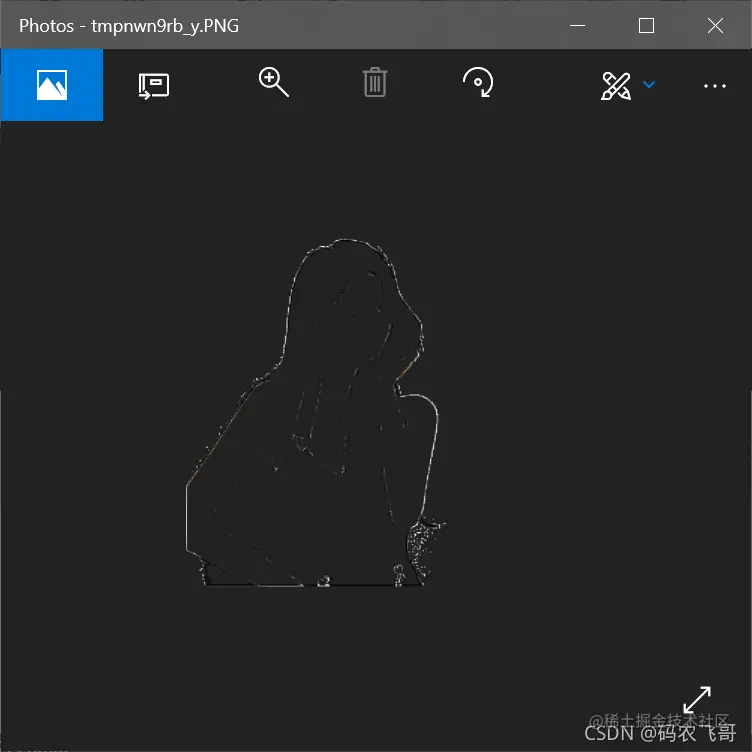6. Relief

``````im5 = im.filter(ImageFilter.EMBOSS)
im5.show()
Copy code ``````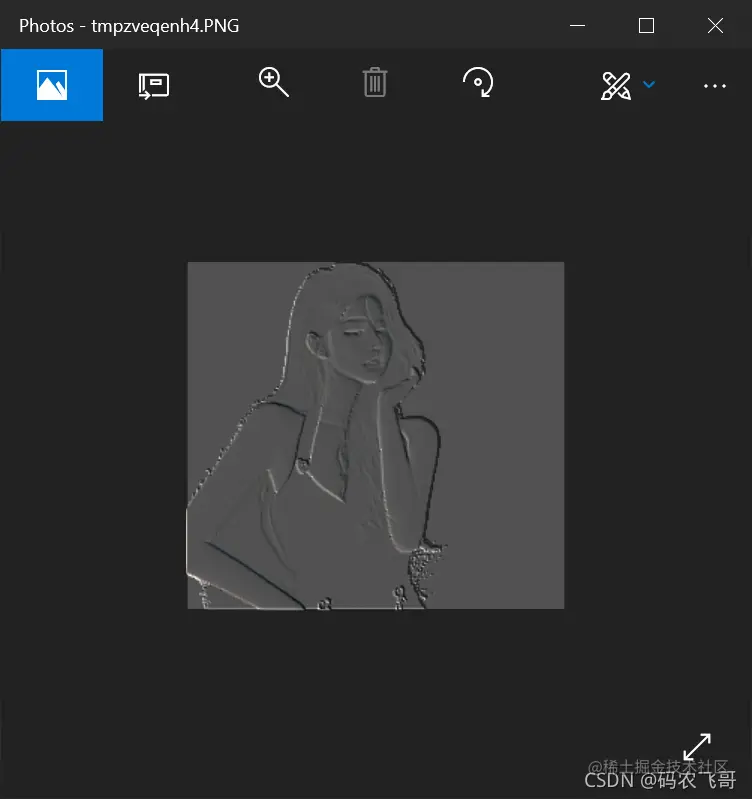7. outline

``````im6 = im.filter(ImageFilter.CONTOUR)
im6.show()
Copy code ``````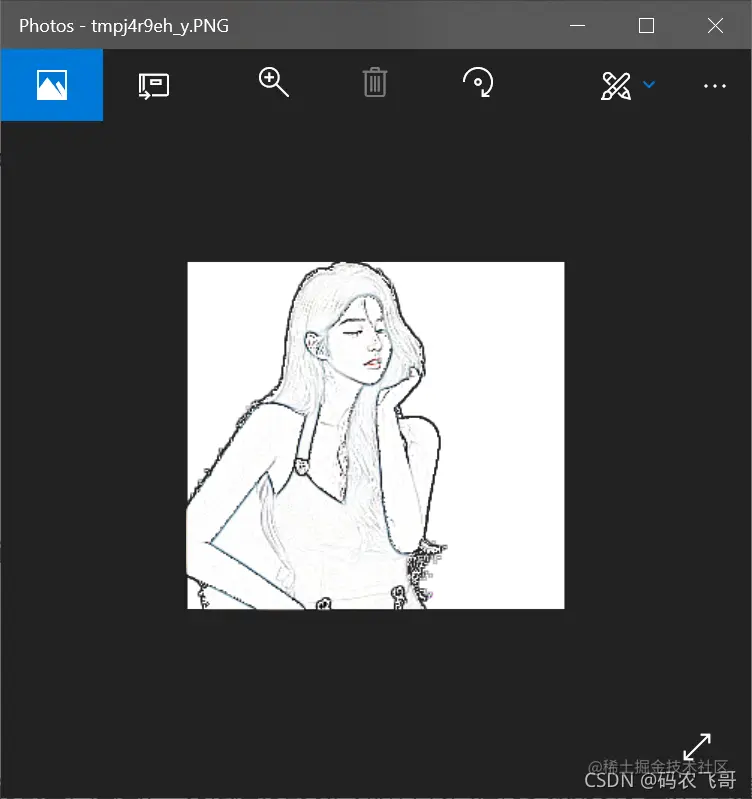8. sharpening

``````im7 = im.filter(ImageFilter.SHARPEN)
im7.show()
Copy code ``````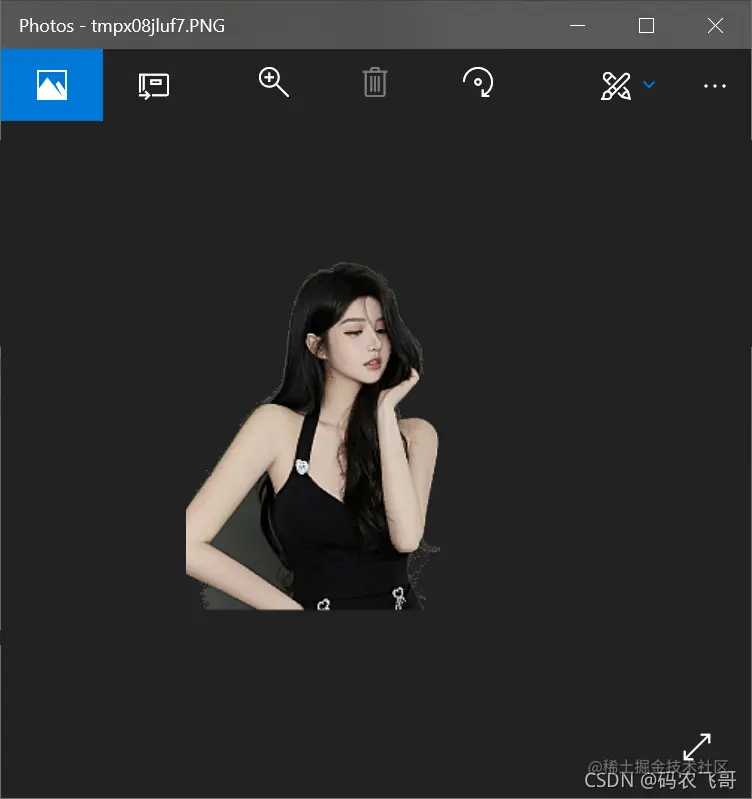## ImageGrab modular

ImageGrab The module is mainly used to take screenshots of the screen , adopt grab Method to intercept , If no parameters are passed in, it means a full screen shot , Otherwise, the image of the specified area is intercepted . among box The format is ：(x1,x2,y1,y2)

``````from PIL import ImageGrab
im1 = ImageGrab.grab((0, 0, 600, 300))  #  Interception screen 600*300 An image of the region of the
im2 = ImageGrab.grab()  #  A full screen capture without parameters
im1.show()
im2.show()
Copy code ``````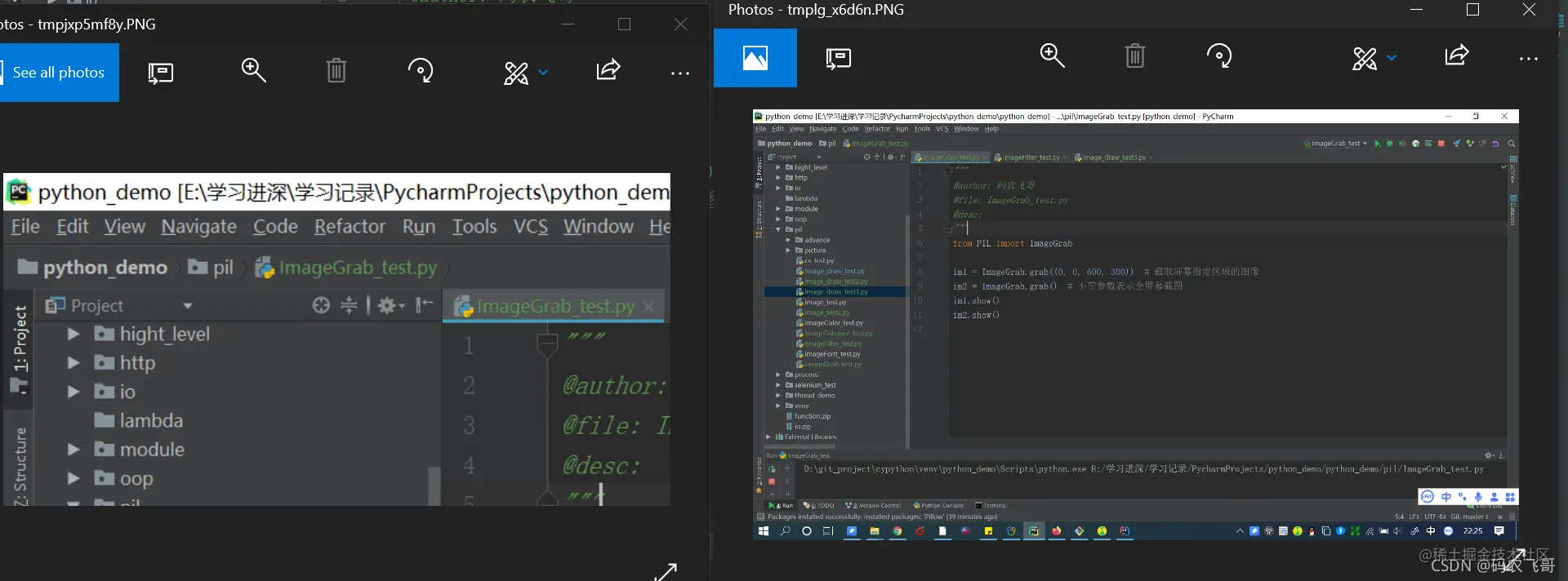## utilize Pillow The library adds watermark to the image

utilize Pillow The library can easily add watermark to the image First , use PIL Of Image Function to read pictures next , Create a new picture ( The size is the same as the original drawing ) then , Use... On the new image PIL Of ImageDraw Draw the words , The color of the word is obtained from the original drawing .

``````from PIL import Image, ImageDraw, ImageFont

font_size = 8
text = " Pool beauty "

img_raw = Image.open("../picture/beautiful.jpeg")
# 1. Read images , Get each pixel value
# 2.  New canvas , Choose the font size to use
new_img = Image.new('RGB', img_raw.size, 'gray')
font = ImageFont.truetype("../picture/simsun.ttf", size=font_size)
draw = ImageDraw.Draw(new_img)

#  Build a generator （ Keep cycling " Pool beauty "）
def character_generate(text):
while True:
for i in range(len(text)):
yield text[i]

char_gen = character_generate(text)
#  Realization effect
for y in range(0, img_raw.size, font_size):
for x in range(0, img_raw.size, font_size):
draw.text((x + 1, y + 1), next(char_gen), font=font, fill=img_array[x, y])

new_img.convert('RGB').save("../picture/beautiful_result.jpeg")
Copy code ``````

Original picture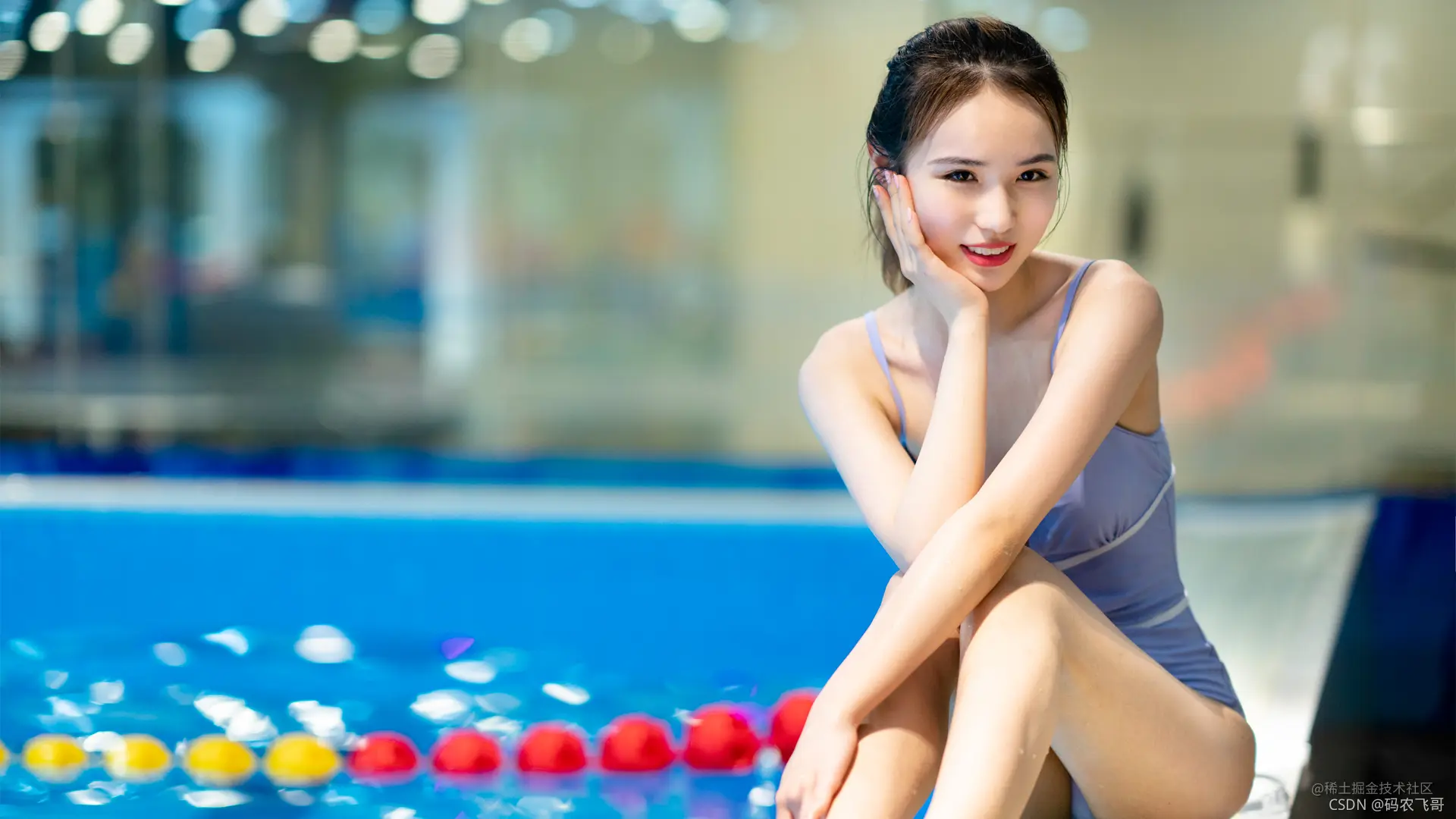Effect picture after adding text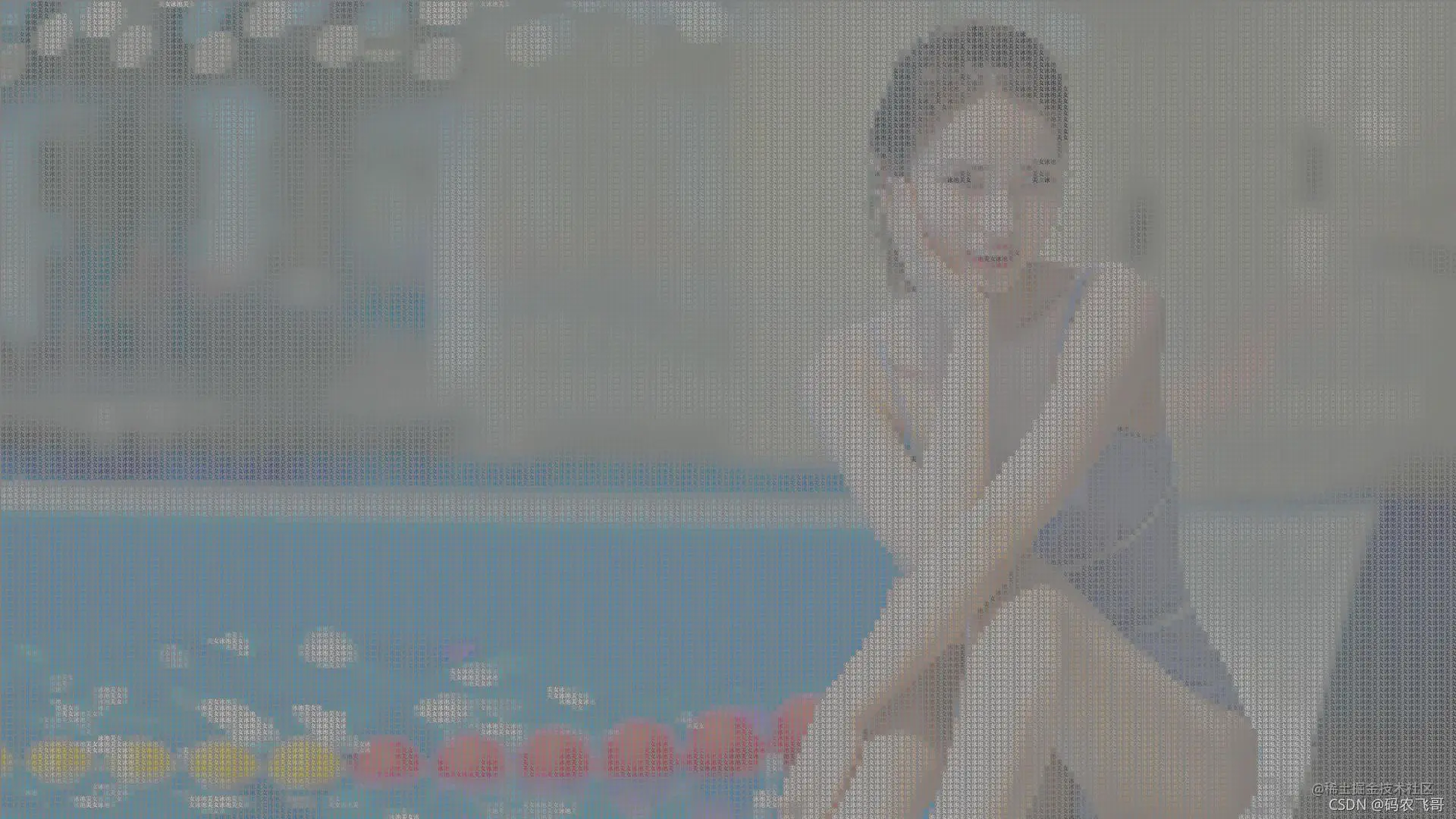## Reference resources

Pillow Official documents

## The source code for

The buddy who needs to get the source code can pay attention to the official account below , reply 【python】

I'm Manon Feige , Thank you again for reading this article . The whole network has the same name 【 Manon Feige 】. Short step , A thousand miles , Enjoy sharing I'm Manon Feige , Thank you again for reading this article .